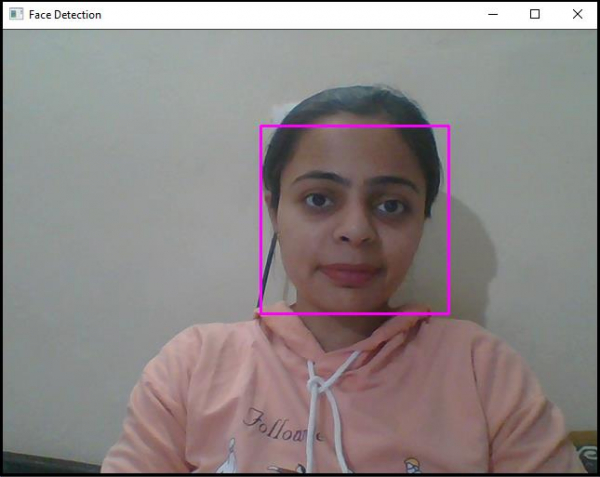# How to detect human faces in real-time in OpenCV using C++?

Detecting face in real-time is similar to detecting a face in still pictures.  The only difference is in real-time face detection, and we have to take a video stream of computer. In this program, we used 'VideoCapture()' function. This function captures video from other camera and stores the frames temporarily in matrix assigned to it. Here this function captures video from the default camera and temporarily stores the frames in 'real_time' matrix.

The following program detects human faces in real-time −

## Example

#include<iostream>
#include<opencv2/highgui/highgui.hpp>
#include<opencv2/imgproc/imgproc.hpp>
//This header includes definition of 'rectangle()' function//
#include<opencv2/objdetect/objdetect.hpp>
#include<string>
using namespace std;
using namespace cv;
int main(int argc, char** argv) {
Mat video_stream;//Declaring a matrix hold frames from video stream//
VideoCapture real_time(0);//capturing video from default webcam//
namedWindow("Face Detection");//Declaring an window to show the result//
string trained_classifier_location = "C:/opencv/sources/data/haarcascades/haarcascade_frontalface_alt.xml";//Defining the location our XML Trained Classifier in a string//
vector<Rect>faces;//Declaring a rectangular vector named faces//
while (true) {
faceDetector.detectMultiScale(video_stream, faces, 1.1, 4, CASCADE_SCALE_IMAGE, Size(30, 30));//Detecting the faces in 'image_with_humanfaces' matrix//
for (int i = 0; i < faces.size(); i++){ //for locating the face
Mat faceROI = video_stream(faces[i]);//Storing face in the matrix//
int x = faces[i].x;//Getting the initial row value of face rectangle's starting point//
int y = faces[i].y;//Getting the initial column value of face rectangle's starting point//
int h = y + faces[i].height;//Calculating the height of the rectangle//
int w = x + faces[i].width;//Calculating the width of the rectangle//
rectangle(video_stream, Point(x, y), Point(w, h), Scalar(255, 0, 255), 2, 8, 0);//Drawing a rectangle using around the faces//
}
imshow("Face Detection", video_stream);
//Showing the detected face//
if (waitKey(10) == 27){ //wait time for each frame is 10 milliseconds//
break;
}
}
return 0;
}

## Output